## Saturday, June 11, 2011

### Irodov Problem 4.30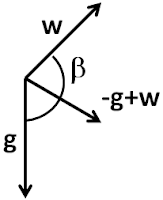The effects on the pendulum will be exactly as the net gravity acting on it were the sum of the vectors -g (upward direction is assumed to be positive) and w i.e. equal to g-w. The magnitude of these vectors is given by,Thus, the time period is thus given by,### Irodov Problem 4.29Consider an instant of time when the body is located at a distance r from the center. Let the density of Earth be p. Let the mass of the body be m. The force F of gravity acting on the body will be proportional to the mass of Earth contained within the sphere of radius r, given by,

We also know that at the surface of the Earth the acceleration is g, hence we have,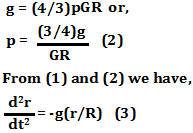(3)
is the equation of motion of the body and is an example of simple harmonic motion. We know that the time period of oscillation is given by,

The time taken by the body to reach the other side of the Earth is then given by,

## Friday, October 22, 2010

### Irodov Problem 4.28First let us consider by understanding all the forces acting on the rod. The rod experiences five forces - i) the two normal reactions N1 and N2 from each of the two rotating wheels ii) the forces of friction kN1 and kN2 acting in a direction so as to oppose the relative motion between the rotatig wheel and the rod and iii) the force of gravity mg the pulls the rod down.

Considering the forces on the rod in the vertical direction we have,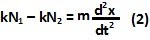In (2), m is the mass of the rod and x the location of the CG of the rod. The location of the CG at the equilibrium position at the center of the wheels is considered to be the origin.

Now let us consider the torques acting on the rod due to the five forces about the CG of the rod. Since the forces of gravity and those due to friction pass through the CG of the rod, they impose no orque on the rod. The two normal reactions from the wheel however do generate torques on the rod. Let l be the distance between the wheels' centers. When the rod's CG is located at x, the distance between the point of contact of the left wheel and the rod and the rod's CG is given by l/2+x. The distance between the point of contact of the right wheel and the rod and the rod's CG is given by, l/2 - x. Thus, the net torque is given by,

Since the rod does not rotate and has no angular acceleration, then net torque on the rod must be zero i.e. T=0.

From (2) and (4) we have,

From, (5) the time period can be computed as,## Wednesday, October 20, 2010

### Irodov Problem 4.27Let h0 be the height of the fluid at equilibrium. Suppose that on the left side the fluid height is depressed by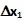then since the total volume of the fluid in the tube should remain the same at any time, fluid height on the right side of the tube will rise. Let the rise in fluid height on the right side be. Since the area of cross section of the tube is S, the area of the horizontal cross section of the inclined part of the tube tube on the right side will be,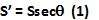The volume of fluid displaced on the left side of the tube is given by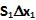and the same of the right side of the tube is given by. Since the total volume of the liquid must be conserved we have,

Now let us consider the restoring forces acting on the fluid. Let S be the cross section of the tube at the mid-point of the tube in the bottom part. Assuming the height of the liquid h0 is much larger than the radius of the tube and the variation of pressure across the crosssection of the tube is negligible, the force that the left side of the fluid exerts on the right side of the fluid is given by,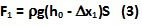Similarly, the force that the right side of the fluid exerts on the left side of the fluid is given by,

The net restoring force on the fluid will thus be,

Hence, from Newton's laws of motion we have,

From (6) it is clear that the time period of oscillations is given by,## Sunday, July 4, 2010

### Irodov Problem 4.26As shown in the figure the perpendicular component of tension in each half of the string will result in the restoring force that acts on the mass. Hence, we have,### Irodov Problem 4.25Let l1 and l2 be the rest lengths of the two springs. When the mass is suspended, it will cause the springs to elongate and support the hanging mass. when the springs + mass system is in equilibrium, let the elongations of the two springs be x1 and x2. The total elongation of the two springs put together will be,Perhaps the most important concept to understand in solving this problem is to understand that the tension in both the springs must be exactly the same if the mass of the springs is zero. To understand why, let us assume that each of the springs have different tensions T1 and T2. Then, the net force acting on the section AB will be T1 - T2. Since the mass of this section is zero T1 must be equal to T2 since otherwise the section will undergo an infinite acceleration.

At equilibrium,There are two forces acing on the mass i) the tension in the spring T2 and ii) the force of gravity mg. Since the mass is at equilibrium,Now consider the state when the mass is pulled a distancefrom its equilibrium position. Let the respective elongations for the springs from their equilibrium positions beand. Then we have,Using the same argument as before,Now the equation of motion of the mass can be written as,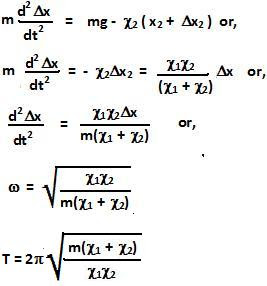### Irodov Problem 4.24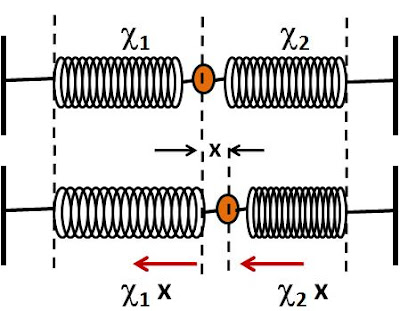When the particle is pushed to the right, the right spring gets compressed while the left spring expands. The net force acting on the particle when the particle is pushed a distance x to the right is given by,